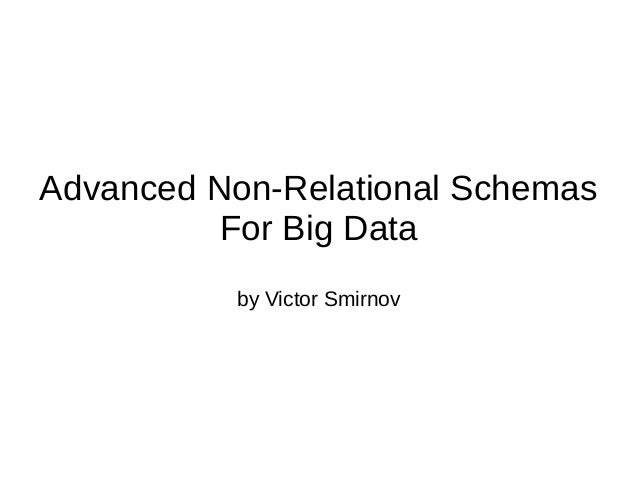Successfully reported this slideshow.
We use your LinkedIn profile and activity data to personalize ads and to show you more relevant ads. You can change your ad preferences anytime.Upcoming SlideShare
×

# Advanced Non-Relational Schemas For Big Data

6,813 views

Published on

This is the presentation from barcamp in Altoros where I was explaining how various advanced non-relational schemas (or, simply, data structures) can be modelled on top of Key/Value storage. The set of covered schemas includes Dynamic Vector, File System, Searchable Bitmap, LOUDS Tree, Wavelet Tree and Inverted Index.

See https://bitbucket.org/vsmirnov/memoria/wiki/MemoriaForBigData

Published in: Data & Analytics
• Full Name
Comment goes here.

Are you sure you want to Yes No
Your message goes here• Be the first to comment

### Advanced Non-Relational Schemas For Big Data

1. 1. Advanced Non-Relational Schemas For Big Data by Victor Smirnov
2. 2. Non-Relational Schema ● Is just a data structure ● That uses some Memory Model ● Typically, Key->Value mapping ● Where Key is an Integer ID ● And Value is an arbitrary array of a limited size or memory block ● It's assumed that operations on memory blocks are atomic.
3. 3. Storage Options
4. 4. Partial (Prefix) Sums Tree ● Given a sequence of S[0, N) = s0...sn-1 of non- negative integers ● Sum(i) returns X = s0+s1+...+si. ● FindLT(X) returns position i of largest Sum(i) < X ● FindLE(X) is the same, but Sum(i) <= X ● We can also define range versions of Sum(i, j) and FindLT(j, X) ● All operations perform in O(log N) time.
5. 5. Packing Perfect Balanced Tree into an Array
6. 6. Some Performance Bits 0 5e+06 1e+07 1.5e+07 2e+07 2.5e+07 3e+07 3.5e+07 4e+07 4.5e+07 5e+07 1 4 16 64 256 1024 4096 16384 65536 262144 Performance,operations/sec Memory Block Size, Kb PackedTree random read performance, 1 million random reads PackedTree<BigInt>, 2 children PackedTree<BigInt>, 32 children std::set<BigInt>, 2 children L1 L2 L3 RAM
7. 7. Dynamic Vector ● An ordered sequence of elements (bytes, integers, strings) of size N ● Acess(i) is O(log N) ● Insert(i, value) is O(log N) ● Delete(i) is O(log N) ● We can also define batch operations: ● Insert(i, value[]) ● Delete(i, j) ● Split(i); Merge(AnotherVector);...
8. 8. Dynamic Vector
9. 9. Dynamic Vector Operations ● FindLT(i) returns the B where i bounds and offset j in the block B for i ● Acces(i) is O(log N) ● Insert(i, value) and Delete(i) are also O(log N) because the tree is balanced.
10. 10. File System: Map<ID, Vector<T>> ● Maps ID to Vector<T> ● Merge all values into one large Dynamic Vector, in ID order ● Create separate “index” sequence from pairs <ID, Offset> in ID order ● We can represent this “index” sequence as two partial sums tree, for ID and for Offset ● We can merge both these trees to one because they have exactly the same structure: multi-index balanced partial sums tree.
11. 11. Map<ID, Vector<T>>
12. 12. Sharing Tree Structures ● Tree structure sharing saves both space and time: SPMD principle (single program, multiple data) ● We can align partial sum trees with different structures using interpolation (padding with zeroes) ● We can merge index and data streams (index and data) of Map<ID, Vector<T>> in one multi-stream tree. ● Merging the trees, we will try to fix index pairs and corresponding data into the same leaf node of multi- stream tree.
13. 13. Multistream Tree Node Layout
14. 14. Multistream Balanced Tree
15. 15. ACID ● Atomic block operations are not enough ● Even simple tree update affects several blocks ● So, ACID is mandatory for advanced non- relational schemas ● We can get ACID for free with Multi-Version Concurrency Control (MVCC) ● We need Version History over data blocks ● Where each each transaction is a version.
16. 16. Transaction History via MVCC
17. 17. Version History Implementation ● Version History maps pair <ID, Version> to an ID of real data block for that version and given ID ● We have Map<ID, Vector<Version, ID>> ● We can turn it to Version History by sorting each Vector<Version, ID> (less sapce, slower) ● Or by creating additional partial sums tree index on top of it (more space, but much faster) ● We can do it in just one multi-stream balanced tree ● MVCC requires some other data structures but they can be designed by analogy.
18. 18. Concurrency Handling ● Version History is a complicated data structure ● Concurrent access to it must be restricted ● Split whole Version History to shards ● And shard blocks by ID to reduce lock contention on Version History
19. 19. Distributed Storage and Processing ● MVCC is very Raft/Paxos-friendly ● Because of Version History and MVCC ● So we can join storage nodes to Raft groups ● And join Raft groups to larger groups with 2PC ● Using split/merge model to map data to nodes.
20. 20. Bonus Slides
21. 21. Searchable Bitmaps ● rank1(n) = number of ones in [0, n) ● select1(i) = position of i-th 1 in the bitmap ● rank0(n) = number of zeroes in [0, n) ● select0(i) = position of i-th 0 in the bitmap
22. 22. Searchable Bitmap: Structure
23. 23. Searchable Bitmaps: Views
24. 24. LOUDS Tree
25. 25. LOUDS Tree: Parent()
26. 26. Wavelet Tree ● Searchable sequence [0...N) for large alphabets ● Rank(i, s) returns number of symbols s in [0, i) ● Select(k, s) returns position i of k-th symbol s ● Insert(i, s), Delere(i), Access(i) – insert, remove and access the symbol at position i respectively ● All these operations have O(log N) time complexity ● By mapping numbers to symbols we can perform the following lookup operations: >, >=, <, <=, <> in O(log N) time.
27. 27. Wavelet Tree: Structure
28. 28. Wavelet Tree: Rank
29. 29. Wavelet Tree: Inverted Index
30. 30. Inverted Index Lookup
31. 31. Thanks! More details are at: https://bitbucket.org/vsmirnov/memoria/wiki/MemoriaForBigData